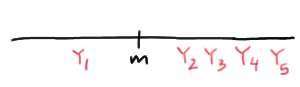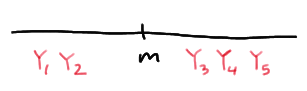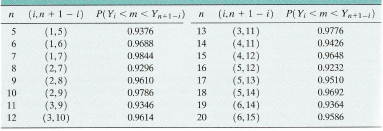# Lesson 19: Distribution-Free Confidence Intervals for Percentiles

Lesson 19: Distribution-Free Confidence Intervals for Percentiles

## Overview

In the previous lesson, we learned how to calculate a sample percentile as a point estimate of a population (or distribution) percentile. Just as it is a good idea to calculate confidence intervals for other population parameters, such as means and variances, it would be a good idea to learn how to calculate a confidence interval for percentiles of a population. That's what we'll work on doing in this lesson. As the title of the lesson suggests, we won't make any assumptions about the distribution of the data, that is, other than it being continuous.

## Objectives

Upon completion of this lesson, you should be able to:

• To learn how to calculate a confidence interval for a median using order statistics.
• To learn how to calculate a confidence interval for any population percentile using order statistics.

# 19.1 - For A Median

19.1 - For A Median

## The Method

As is generally the case, let's motivate the method for calculating a confidence interval for a population median $$m$$ by way of a concrete example. Suppose $$Y_1<Y_2<Y_3<Y_4<Y_5$$ are the order statistics of a random sample of size $$n=5$$ from a continuous distribution. Our work from the previous lesson tells us that $$Y_3$$ serves as a good point estimator of the median $$m$$. Let's see what we can come up with for a confidence interval given we have these order statistics at our disposal. Well, suppose we suggested that the interval constrained by the first and fifth order statistics, that is, $$(Y_1, Y_5)$$ would serve as a good interval. How confident can we be that the interval $$Y_1, Y_5)$$ would contain the unknown population median $$m$$? To answer that question, we simply need to calculate the following probability:

$$P(Y_1<m<Y_5)$$

Calculating the probability reduces to a simple binomial calculation once we figure out all the ways in which the population median $$m$$ is sandwiched between $$Y_1$$ and $$Y_5$$. Well, the population median m is sandwiched between $$Y_1$$ and $$Y_5$$, if the first order statistic is the only order statistic less than the median $$m$$:The population median $$m$$ is sandwiched between $$Y_1$$ and $$Y_5$$, if the first two order statistics are the only order statistics less than the median $$m$$:The population median $$m$$ is sandwiched between $$Y_1$$ and $$Y_5$$, if the first three order statistics are less than the median $$m$$, and the fourth and fifth order statistics are greater than $$m$$:And, the population median $$m$$ is sandwiched between $$Y_1$$ and $$Y_5$$, if the fifth order statistic is the only order statistic greater than the median $$m$$:This means that in order to calculate the probability $$P(Y_1<m<Y_5)$$, we need to calculate the probability of each of the above events. Now, if we let $$W$$ denote the number of $$X_i<m$$, then $$W$$ is a binomial random variable with $$n$$ mutually independent trials and probability of success $$p=P(X_i<m)=0.5$$. And, reviewing the events as depicted above, the desired probability is calculated as:

$$P(Y_1<m<Y_5)=P(W=1)+P(W=2)+P(W=3)+P(W=4)$$

The binomial p.m.f. (or, alternatively, the binomial table) makes the calculation straightforward:

$$P(Y_1<m<Y_5)=\sum_{k=1}^{4}P(W=k)=\sum_{k=1}^{4}\binom{5}{k}(0.5)^k(0.5)^{5-k}=0.9376$$

So, the probability that the random interval $$(Y_1, Y_5)$$ contains the median $$m$$ is 0.9376. We aren't always so lucky with arriving at a decent confidence coefficient on our first try. Sometimes we have to try again aiming to get a confidence coefficient that it as least 90%, but as close to 95% as possible. In this case, the confidence coefficient for the interval $$(Y_2, Y_4)$$ is:

$$P(Y_2<m<Y_4)=\sum_{k=2}^{3}P(W=k)=\sum_{k=2}^{3}\binom{5}{k}(0.5)^k(0.5)^{5-k}=0.6250$$

Clearly, we would be better served to stick with the interval $$(Y_1, Y_5)$$ in this case. Let's take a look at an example.

## Example 19-1An ecology laboratory studied tree dispersion patterns for the sugar maple whose seeds are dispersed by the wind. In a 50-meter by 50-meter plot, the laboratory researchers measured distances between like trees yielding the following distances, in meters and in increasing order, for 19 sugar maple trees:

2.10 2.35 2.35 3.10 3.10 3.15 3.90 3.90 4.00 4.80
5.00 5.00 5.15 5.35 5.50 6.00 6.00 6.25 6.45

Find a reasonable confidence interval for the median.

Because there are $$n=19$$ data points, $$y_{10}=4.80$$ serves as a good point estimator for the population median m. Let's go up and down a few spots from there to consider:

$$(y_6, y_{14})=(3.15, 5.35)$$

as a possible confidence interval for $$m$$. The confidence coefficient associated with the interval $$(Y_6, Y_{14})$$ is calculated using a binomial table with $$n=19$$ and $$p=0.5$$:

$$P(Y_6<m<Y_{14})=P(6\le W \le 13)=P(W \le 13) -P(W \le 5)=0.9682-0.0318=0.9364$$

We can therefore be 93.64% confident that the population median falls in the interval (3.15, 5.35).

Could we do any better? Well, if we were to use the narrower interval $$(y_7, y_{13})=(3.90, 5.15)$$ instead, its confidence coefficient is not quite as good:

$$P(Y_7<m<Y_{13})=P(7\le W \le 12)=P(W \le 12) -P(W \le 6)=0.9165-0.0835=0.8330$$

Or, if we were to use the wider interval $$(y_5, y_{15})=(3.10, 5.50)$$ instead, its confidence coefficient is perhaps a bit too high:

$$P(Y_5<m<Y_{15})=P(5\le W \le 14)=P(W \le 14) -P(W \le 4)=0.9904-0.0096=0.9808$$

In general, we should aim to get a confidence coefficient at least 90%, but as close to 95% as possible. And, we shouldn't really "shop around" for an interval after we've collected the data. We should decide in advance which confidence interval we are going to use, and commit to use it even after the data have been collected.

Yeehaw! The authors of your textbook did a very kind thing for us by calculating the confidence coefficients for confidence intervals for the median $$m$$ for various sample sizes $$n$$. The resulting confidence coefficients are reported in the following table (or you can look in your text book if you don't want to use a magnifying glass to see this one):The reading of the table is pretty straightforward. For example, if we have a sample of size $$n=12$$, the table tells us we can be 96.14% confident that the population median falls in the interval constrained by the third and tenth order statistic, that is, in the interval $$(Y_3, Y_{10})$$. And, if we have a sample of size $$n=18$$, the table tells us we can be 96.92% confident that the population median falls in the interval constrained by the fifth and fourteenth order statistic, that is, in the interval $$(Y_5, Y_{14})$$.

## Normal Approximations of the Confidence Coefficients

All of our confidence coefficient calculations have involved binomial probabilities. It stands to reason, then, that if our sample size $$n$$ is larger than 20, say, we could use the normal approximation to the binomial distribution. In our case, $$W$$, the number of $$X_i<m$$, follows a binomial distribution with mean and variance:

$$\mu=np=0.5n$$ and $$\sigma^2=np(1-p)=0.5(1-0.5)n=0.25n$$

respectively. Therefore:

$$Z=\frac{W-0.5n}{\sqrt{0.25n}}$$

follows, at least approximately, the standard normal $$N(0,1)$$ distribution.

## Example 19-2A sample of 26 offshore oil workers took part in a simulated escape exercise, resulting in the following data on time (in seconds) to complete the escape:

325 325 334 339 356 356 359 359 363
364 364 366 369 370 373 373 374 375
389 392 393 394 397 402 403 424

Use the normal approximation to the binomial to find the approximate confidence coefficient associated with the $$(Y_8, Y_{18})$$ confidence interval for the median $$m$$. (The data are from the journal article "Oxygen Consumption and Ventilation During Escape from an Offshore Platform," Ergonomics 1997: 281-292.)

In this case, the mean and variance are:

$$\mu=np=0.5(26)=13$$ and $$\sigma^2=np(1-p)=0.5(1-0.5)n=0.25(26)=6.5$$

respectively. Therefore, the approximate confidence coefficient for the interval $$(Y_8, Y_{18})$$ is:

$$P(Y_8<m<Y_{18})=P(8 \le W \le 17)=P\left(\frac{7.5-13}{\sqrt{6.5}} < Z < \frac{17.5-13}{\sqrt{6.5}} \right)$$

which can be simplified to:

$$P(Y_8<m<Y_{18})=P(-2.16 \le Z \le 1.77)=0.9616 - 0.0154 = 0.9462$$

We can be approximately 94.6% confident that the median time of all escapes is between 359 and 375 seconds.

# 19.2 - For Any Percentile

19.2 - For Any Percentile

The method that we learned for finding a confidence interval for the median of a continuous distribution can be easily extended so that we can find a confidence interval for any percentile $$\pi_p$$. The only thing we have to change is the probability of a success, that is, that $$X_i$$ is less than $$\pi_p$$:

$$p=P(X_i < \pi_p)$$

Then, the exact confidence coefficient is calculated just as before using the binomial distribution with parameters $$n$$ and $$p$$:

$$1-\alpha=P(Y_i < \pi_p < Y_j)=\sum_{k=i}^{j-1}\binom{n}{k}p^k(1-p)^{n-k}$$

And, for large samples of size $$n\ge 20$$, say, an approximate confidence coefficient is calculated using the normal approximation to the binomial by way of the standard normal random variable:

$$Z=\dfrac{W-np}{\sqrt{np(1-p)}}$$

Once the sample is observed and the order statistics determined, then the known interval $$(y_i, y_j)$$ serves as a $$100(1-\alpha)\%$$ confidence interval for the unknown population percentile $$\pi_p$$. Let's revisit an example from the previous page.

## Example 19-2 (continued)A sample of 26 offshore oil workers took part in a simulated escape exercise, resulting in the following data on time (in seconds) to complete the escape:

325 325 334 339 356 356 359 359 363
364 364 366 369 370 373 373 374 375
389 392 393 394 397 402 403 424

Find a confidence interval for the 75th percentile, and calculate its confidence coefficient. (The data are from the journal article "Oxygen Consumption and Ventilation During Escape from an Offshore Platform," Ergonomics 1997: 281-292.)

Since $$(0.75)(26+1)=20.25$$, the weighted average of the 20th and 21st order statistic:

$$\tilde{\pi}_{0.75}=y_{20}+0.25(y_{21}-y_{20})=0.75y_{20}+0.25y_{21}=0.75(392)+0.25(393)=392.25$$

serves as a good point estimate of $$\pi_{0.75}$$. To find a confidence interval for $$\pi_{0.75}$$, let's move up and down a few order statistics from $$y_{20}$$ to, say, $$y_{16}$$ and $$y_{24}$$. In that case, our interval is $$(y_{16}, y_{24}=(373, 402)$$ with an exact confidence coefficient calculated using a binomial distribution with n = 26 and p = 0.75 as:

$$P(Y_{16}<m<Y_{24})=P(16 \le W \le 23)=P(W \le 23)-P(W \le 15)=0.9742-0.0401=0.9341$$

We can be 93.4% confident that the 75th percentile of all escape times is between 373 and 402 seconds.

Because $$n=26$$ here, we could have alternatively used the normal approximation to the binomial. In this case, the mean and variance are:

$$\mu=np=0.75(26)=19.5$$ and $$\sigma^2 =np(1-p)=26(0.75)(1-0.75)=4.875$$

respectively. Therefore, the approximate confidence coefficient for the interval $$(y_{16}, y_{24})$$ is:

$$P(Y_{16}<m<Y_{24})=P(16 \le W \le 23)=P\left(\dfrac{15.5-19.5}{\sqrt{4.875}} < Z < \dfrac{23.5-19.5}{\sqrt{4.875}} \right)$$

which can be simplified to:

$$P(Y_{16}<m<Y_{24})=P(-1.81 \le Z \le 1.81)=0.9649-0.0359=0.929$$

As you can see, the normal approximation does quite well, as the approximate probability is 0.929 compared to the exact probability of 0.934.

  Link ↥ Has Tooltip/Popover Toggleable Visibility